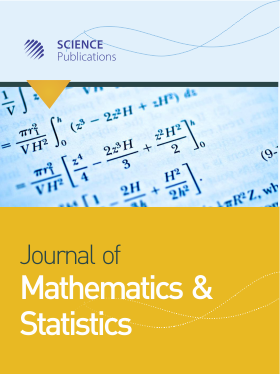Frequency: Continuous
ISSN: 1549-3644 (Print)
ISSN: 1558-6359 (Online)
Research Article Open Access

# A Distribution-Free Bayesian Approach for Indirect Comparisons

• Johanna S. van Zyl Baylor University, United States
• Jack D. Tubbs Baylor University, United States

Journal of Mathematics and Statistics
Volume 15 No. 1, 2019, 136-145
DOI:

## Abstract

The objective of this paper is to consider an indirect comparison between treatments B and C when each have been compared directly to treatment A in separate studies. Problems of this type are common in Network Meta-Analysis (NMA). A commonly used method assumes that the underlying data, Y, are normally distributed and µ = E(Y) is the measure of clinical effectiveness. The normal assumption is often violated. In addition, the sample sizes are not necessarily large. These conditions challenge the concept that a single location parameter, such as, the mean or median should be used as the measure of clinical effectiveness in the analysis. In this paper, we present an alternative approach where the Area Under the ROC Curve (AUC) is used as the measure of clinical effectiveness. Since the normal distribution may be uncertain, we use a distribution-free Bayesian mixtures of Finite Polya Trees (MFPT) model with the AUC in order to make the indirect comparison.

### How to Cite

van Zyl, J. S. & Tubbs, J. D. (2019). A Distribution-Free Bayesian Approach for Indirect Comparisons. Journal of Mathematics and Statistics, 15(1), 136-145. https://doi.org/10.3844/jmssp.2019.136.145